### Chemical Kinetics Class 12th Chemistry Part I CBSE Solution

##### Question 1.From the rate expression for the following reactions, determine their order of reaction and dimensions of the rate constants.(i) 3NO(g) → N2O (g) Rate = k[NO]2(ii) H2O2 (aq) + 3I–(aq) + 2H+→ 2H2O(l) + I–3 Rate = k[H2O2][I–](iii) CH3CHO(g) → CH4(g) + CO(g) Rate = k[CH3CHO]3/2(iv) C2H5Cl (g) → C2H4(g) + HCl (g) Rate = k[C2H5Cl]Order is sum of all powers of the concentrations of the reactant in “rate law expression”Answer:(i) 3NO(g) → N2O(g)Rate = k[NO]2Here rate law is dependent on concentration of NO only.And power raised to concentration of NO is 2. Hence order of reaction is 2.To find dimensions of rate constant (K)- We know rate of chemical reaction is measured in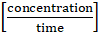. So, unit of K will be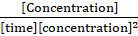i.e. [concentration]-1[ time]-1 . If concentration is in mol L-1 and time is in sec. then dimensions of k will be : mol-1 L sec-1(ii) H2O2(aq) + 3I-(aq) + 2H+ → 2 H2O(l) + I3-Rate = k[H2O2][I-]Here rate law is dependent on concentration of H2O2 and I-. And power raised to concentration of H2O2 is 1, and power raised to concentration of I- is 1. order is sum of powers hence, order of given reaction is 2.To find dimensions of rate constant (K)- We know rate of chemical reaction is measured in [concentration/time]. So, unit of K will be [Concentration]/[time][concentration]2 i.e. [concentration]-1[ time]-1. If concentration is in mol L-1 and time is in sec then dimensions of k will bemol-1 L sec-1(iii) CH3CHO(g) → CH4(g) + CO(g)Rate = k[CH3CHO]3/2Here rate law is dependent on concentration of CH3CHO.And power raised to CH3CHO is 3/2. Hence order of reaction is 3/2.To find dimensions of rate constant (K)- We know rate of chemical reaction is measured in [concentration/time]. So, unit of K will be [Concentration]/[time][concentration]3/2 i.e. [concentration]-1/2[time]-1.If concentration is in mol L-1 and time is in sec. Then dimensions of K will be mol-1/2L1/2 sec-1(iv) C2H5Cl (g) → C2H4(g) + HCl(g)Rate = k[C2H5Cl]Here rate law is dependent on concentration of C2H5Cl. And power raised to C2H5Cl is 1. Hence order of reaction is 1.To find dimensions of rate constant (K)- We know rate of chemical reaction is measured in [concentration/time]. So, unit of K will be [Concentration]/[time][concentration]1. i.e. [time]-1If concentration is in mol L-1 and time is in sec. Then dimensions of K will be sec-1.Question 2.For the reaction :2A + B → A2Bthe Rate = k[A][B]2 with k = 2.0 × 10-6 mol-2 L2 sec-1. Calculate the initial rate of the reaction when [A] = 0.1 mol L-1 and [B] = 0.2 mol L-1. Calculate the rate of reaction after [A] is reduced to 0.06 mol L-1.Answer:a) Rate = k[A][B]2, Rate = 2.0 × 10-6[0.1][0.2]2Rate = 8 × 10-9 mol L-1sec-1b)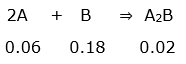Situation when A is remained 0.06 mol L-1Now, According to rate law, Rate = k[A][B]2Rate = 2 × 10-6[0.06][0.18]2i.e. Rate = 3.888 × 10-9 mol L-1sec-1Initial rate of reaction is 8 × 10-9 mol L-1sec-1. and rate when concentration of A is 0.06 mol L-1 is 3.888 × 10-9 mol L-1sec-1.Question 3.The decomposition of NH3 on platinum surface is zero order reaction.What are the rates of production of N2 and H2. if k = 2.5 × 10-4 mol L-1 sec-1?Answer:2NH3(g) → N2(g) + 3H2(g)Rate of zero order reaction is equal to rate constant. i.e. Rate = 2.5 × 10-4mol L-1sec-1.According to rate law,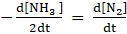2.5 × 10-4mol L-1sec-1 =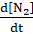i.e. the rate of production of N2 is 2.5 × 10-4mol L-1 sec-1.According to rate law,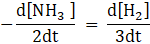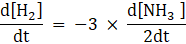i.e. rate of formation of H2 is 3 times rate of reaction = 3 × 2.5 × 10-4mol L-1sec-1= 7.5 × 10-4mol L-1sec-1Rate of formation of N2 and H2 is 2.5 × 10-4 mol L-1sec-1 and 7.5 × 10-4 mol L-1sec-1 respectively.Question 4.The decomposition of dimethyl ether leads to formation of CH4, H2 and CO and the reaction rate is given by,Rate = k[CH3OCH3]3/2. The rate of reaction is followed by an increase in pressure in a closed vessel, so the rate can also be expressed in terms of the partial pressure of dimethyl ether i.e. Rate = k[pCH3OCH3]3/2. If the pressure is measured in bar and time in minutes, then what are the units of rate and rate constants?Answer:Rate of given chemical reaction will be represented as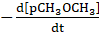.Hence units of rate is bar min-1To find units of K, K = rate/[pCH3OCH3]3/2The unit of k = bar -1/2min-1.Units of Rate- bar min-1. and Units of Rate constant K : bar -1/2min -1Question 5.Mention the factors that affect the rate of chemical reaction?Answer:Factors affecting rate of reaction-1) Temperature (increasing temperature increases rate of reaction)2) Concentration or pressure of reactants. (Increasing concentration or pressure increases rate of reaction)3) Presence or absence of a catalyst. (Adding catalyst mostly increases the rate of reaction)4) The surface area of solid reactant. (If reaction is processing over solid reactant then increasing its surface area increases rate of reaction)5) Nature of reactantsQuestion 6.A reaction is second order with respect to a reactant. How is the rate of reaction affected if the concentration is(i) doubled (ii) reduced to halfAnswer:Let suppose reactionA ⇒ B;having rate law, Rate = K [A]2(i) If the concentration of A is doubled then the rate will affect by the square of concentration i.e. rate will become 22 = 4 times.(ii) If the concentration of A is halved then the rate will affect the square of concentration i.e (1/2)2 = 1/4 times.(i) Rate becomes 4 times of initial Rate (ii) Rate becomes 1/4 times of initial RateQuestion 7.What is the effect of temperature on the rate constant of a reaction? How can this effect of temperature on rate constant be represented quantitatively?Answer:Increase in temperature increases the rate constant of a reaction. as we know increase in temperature increases the rate of reaction to satisfy the equation Rate = k [concentration]n where n can be any real number. k have to increase as concentration is almost not changing over small temperature change. Increasing temperature by 100C almost double the rate constant.This can be represented quantitively by the help of arrehinus equation-K = Ae-Ea/RT, where k is rate constant, Ea is activation energy, R is universal gas constant, T is absolute temperature.Question 8.In a pseudo first order hydrolysis of ester in water, the following results were obtained(i) Calculate the average rate of reaction between the time interval 30 to 60 seconds(ii) Calculate the pseudo first-order rate constant for the hydrolysis of the ester.Answer:(i) Average rate of reaction over interval is [change in concentration]/[time taken] i.e.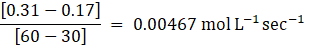(ii) the pseudo first-order rate constant can be calculated byK = (2.303/t) log(Ci/Ct) where K is Rate constant,t is time taken,Ci is initial concentrationCt is Concentration at time t.K = (2.303/30) log (0.55/0.31)⇒ K = 1.9 × 10-2 sec-1(i) Average rate between 30 to 60 sec is 0.00467 mol L-1sec-1(ii) Pseudo first order rate constant is 1. × 10-2sec-1Question 9.A reaction is First Order in A and second order in B.(i) Write the differential rate equation.(ii) How is the rate affected on increasing the concentration of B three times?(iii) How is the rate affected when the concentrations of both A and B are doubled?Answer:(i) Order is power raised to reactant in rate law, hence,Rate = k[A][B]2(ii) When the concentration of B is increased three times then the rate is affected by the square of reactant. The rate is increased by 9 Times.(iii) When concentration of reactant both A and B is doubled then the rate will have affected as square of reactant B and Two times of Reactant A. Overall increase in rate is 8 times(i) When the concentration of B is increased by three times, the rate is increased by nine times.(ii) When conc. of both reactants doubled then Rate increased 8 times.Question 10.In a reaction between A and B, the initial rate of reaction(r0)was measured for different initial concentrations of A and B are given below:What is the order of reaction with respect to A and B?Answer:When concentration of B is changed then rate of reaction doesn't change that means order with respect to B is 0. But when the concentration of A is doubled rate increased by 2.82 times i.e.21.5 = 2.82.Hence order with respect to A is 1.5.Order with respect to A and B is 1.5 and 0 respectively.Question 11.The following results have been obtained during the kinetic studies of the reaction:2A + B → C + DDetermine the Rate law and the rate constant for the reaction.Answer:By comparing Experiment I and IV if we increase the concentration of A by 4 times then Rate also increased by 4 times. That means order with respect to A is 1.By comparing Experiment II and III if we double the concentration of B Rate increases by 4 times that means order with respect to B is 2.Rate law of reaction will be, Rate = k [A][B]2To find K, K = rate/[A][B]2 i.e. K = 6.0 × 10-3/[0.1][0.1]2K = 6 mol-2L2sec-1Order with respect to A and B is 1 and 2 Respectively. And value of K(rate constant) is = 6 mol-2L2sec-1Question 12.The reaction between A and B is first order with respect to A and zero order with respect to B.Phil. in the blanks in the following table:Answer:As reaction is first order with respect to A and zero Order with respect to B. Then changing the concentration of B won’t affect the rate of reaction and increasing concentration of A ‘n’ times will increase the rate by ‘n’ times. By this logic lets fill the table- In first blank space concentration of A will be 0.2 mol L-1 because the rate is doubled. In second blank space, Rate will be 8 × 10-2mol L-1min-1 because the concentration of A is increased 4 Times. In third blank space concentration of A will be 0.1 mol L-1 because the rate is same as in experiment I.Question 13.Calculate the half life of a first order reaction from their rate constants given below:(i) 200 s-1 (ii) 2 min-1 (iii) 4 years-1Answer:Half life of first order reaction is, t1/2 = ln2/K where t1/2 is half life of first order reaction, K is rate constant of First order reaction.(i) t1/2 = ln2/200 s-1⇒ t1/2 = 0.693/200 s-1 (∵ ln2 = 0.693)⇒ t1/2 = 0.003465 sec.(ii) t1/2 = ln2/2 min-1⇒ t1/2 = 0.693/2 min-1 (∵ ln2 = 0.693)⇒ t1/2 = 0.3465 min(iii) t1/2 = ln2/4 year-1⇒ t1/2 = 0.693/4 years -1 (∵ ln2 = 0.693)⇒ t1/2 = 0.17325 year.Half life of 3 reactions are 0.003465 sec, 0.3465 min, 0.17325 year respectively.Question 14.The half-life for radioactive decay of 14C is 5730 years. An archeological artifact containing wood had only 80%of the 14C found in a living tree. Estimate the age of the sample.Answer:Radio active decay occurs via first order rate law,t1/2 = 5730 years. rate constant(k) of given decay is 0.693/t1/2⇒ 0.693/5730 = 1.2 × 10-4 year-1By first order integrated rate law age of sample will be,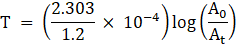where T is the age of sample A0 is the initial activity of the sample. and At is the activity of sample at any time t.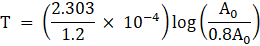T = 0.18 × 104 years.Age of given sample is 0.18 × 104 years.Question 15.The experiemental data for decomposition of N2O5[2N2O5→ 4NO2 + O2]in gas phase at 318 k are given below:(i) plot N2O5 against t(ii) Find the half-life period for the Reaction.(iii) Draw a graph between log[N2O5]and t.(iv) what is the rate law?(v) Calculate the rate constant.(vi) Calculate the half-life period from k and compare it with (ii)Answer: (i)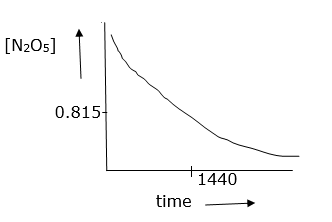(ii) initial conc.is 1.63 × 10-2 so half conc. is 0.815 × 10-2M From given information and graph t1/2 is 1440 sec.(iii)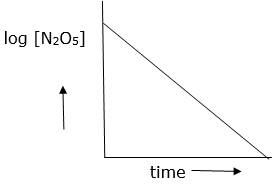(iv) As log [N2O5] vs time is straight line given reaction is first order. Hence its rate law will be, Rate = k[N2O5](v) The slope of above graph is slope = 0.000209K = 2.303 × slope⇒ 4.82 × 10-4sec-1Now, t1/2 = 0.693/K.⇒ 0.693/4.82 × 10-4⇒ t1/2 = 1438 sec. which is almost equal to (ii)Question 16.The rate constant for a first order reaction is 60 s–1. How much time will it take to reduce the initial concentration of the reactant to its 1/16th value?Answer:Given:Order of the reaction = 1Let, Initial concentration [R]°= xFinal concentration [R] = x/16Rate constant k = 60 s-1We know, time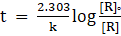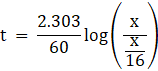⇒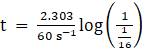⇒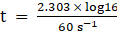Solving, we get t = 4.6 × 10-2sQuestion 17.During nuclear explosion, one of the products is 90Sr with half-life of 28.1 years. If 1μg of 90Sr was absorbed in the bones of a newly born baby instead of calcium, how much of it will remain after 10 years and 60 years if it is not lost metabolically.Answer:Initial concentration, [R]° = 1μgFinal concentration, [R]Half-life t1/2 = 28.1 yrsSolution:We know, t1/2 = 0.693/kWhere, k – rate constant⇒ k = 0.693/ t1/2⇒ k = 0.693/(28.1 yrs)⇒ k = 0.0246 yrs-1Also,If t = 10yrs, then, using the formula, we get,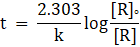⇒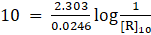⇒⇒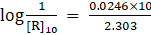⇒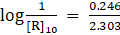⇒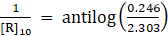⇒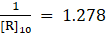⇒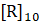= 0.7824μgIf t = 60yrs, then again, we get,⇒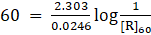⇒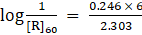⇒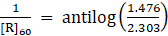⇒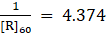⇒ [R]60 = 0.228 μg∴ 0.2278μg of 90Sr will be left after 60 years.Question 18.For a first order reaction, show that time required for 99% completion is twice the time required for the completion of 90% of reaction.Answer:Let, initial concentration be [R]°Concentration at 90% completion be ((100-90)/100)×[R]°∴ Concentration at 90% be 0.1[R]°Concentration at 99% completion be ((100-99)/100)× [R]°∴ Concentration at 99% be 0.01[R]°We know, timeTime taken for 90% completion is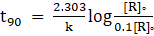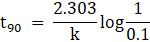⇒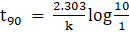⇒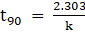Time taken for 99% completion is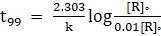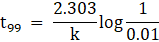⇒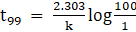⇒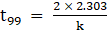⇒ t99 = 2t90Hence, the time taken to complete 9% of the first order reaction is twice the time required for the completion of 90% of the reaction.Question 19.A first order reaction takes 40 min for 30% decomposition. Calculate t1/2.Answer:Given:Time t = 40 minWhen 30% decomposition is undergone, 70% is the concentration.We know, time takenWhere, k- rate constant[R]° -Initial concentration[R]-Concentration at time ‘t’⇒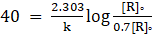⇒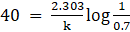⇒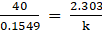⇒ 258.23 = (2.303/k)We know, Half-life t1/2 = 0.693/kWhich can be written as, t1/2 = 0.3010 × (2.303/k)⇒ t1/2 = 0.3010 × 258.23⇒ t1/2 = 77.72 minQuestion 20.For the decomposition of azoisopropane to hexane and nitrogen at 543 K, the following data are obtained.Calculate the rate constant.Answer:When t = 0, the total partial pressure is P0 = 35.0 mm of Hg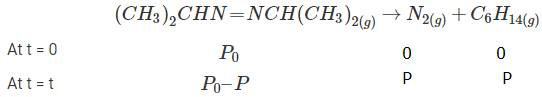When time t = t, the total partial pressure is Pt = P0 + pP0-p = Pt-2p, but by the above equation, we know p = Pt-P0Hence, P0-p = Pt-2( Pt-P0)Thus, P0-p = 2P0 – PtWe know that timeWhere, k- rate constant[R]° -Initial concentration of reactant[R]-Concentration of reactant at time ‘t’Here concentration can be replaced by the corresponding partial pressures.Hence, the equation becomes,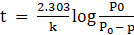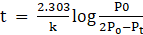→ equation 1At time t = 360 s, Pt = 54 mm of Hg and P0 = 30 mm of Hg,Substituting in equation 1,⇒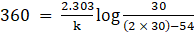⇒ k = 2.175 × 10-3 s-1At time t = 720 s, Pt = 63 mm of Hg and P0 = 30 mm of Hg,Substituting in equation 1,⇒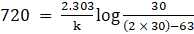Thus, k = 2.235 × 10-3 s–1Taking average, k = (2.235 × 10-3 s–1 + 2.175 × 10-3 s–1)/2∴ k = 2.21 × 10-3 s–1.Question 21.The following data were obtained during the first order thermal decomposition of SO2Cl2 at a constant volume.SO2Cl2(g)→ SO2 (g) + Cl2 (g)Calculate the rate of the reaction when total pressure is 0.65 atmAnswer:When t = 0, the total partial pressure is P0 = 0.5 atm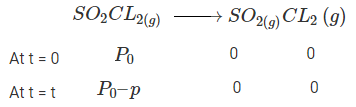When time t = t, the total partial pressure is Pt = P0 + pP0-p = Pt-2p, but by the above equation, we know p = Pt-P0Hence, P0-p = Pt-2(Pt-P0)Thus, P0-p = 2P0 – PtWe know that timeWhere, k- rate constant[R]° -Initial concentration of reactant[R]-Concentration of reactant at time ‘t’Here concentration can be replaced by the corresponding partial pressures.Hence, the equation becomes,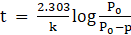⇒→ equation 1At time t = 100 s, Pt = 0.6 atm and P0 = 0.5 atm,Substituting in equation 1,⇒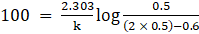Thus, k = 2.231 × 10-3 s-1The rate of reaction R = k × PS02Cl2When total pressure Pt = 0.65 atm and P0 = 0.5 atm, thenPS02Cl2 = 2P0-PtThus, substituting the values, PS02Cl2 = 2(0.5)-0.6 = 0.35 atmR = k × PS02Cl2 = 2.231 × 10-3 s–1 × 0.35Rate of the reaction R = 7.8 × 10-4atm s–1Question 22.The rate constant for the decomposition of N2O5 at various temperatures is given below:Draw a graph between ln k and 1/T and calculate the values of A and Ea. Predict the rate constant at 30° and 50°C.Answer:To convert the temperature in °C to °K we add 273 K.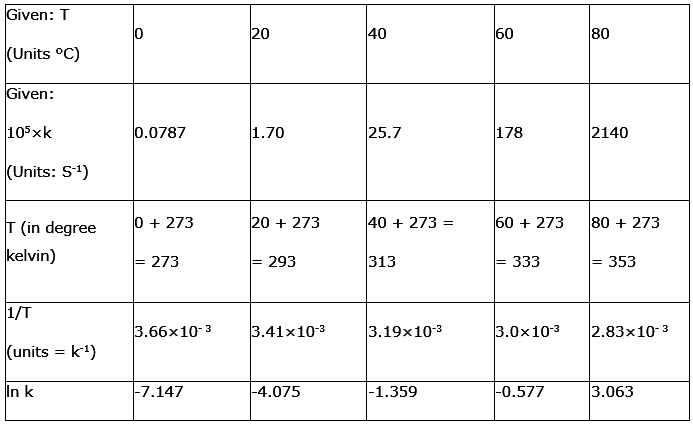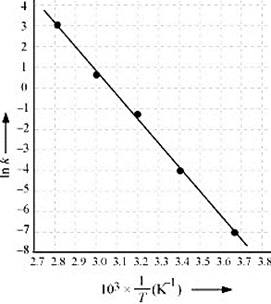The graph is given as:The Arrhenius equation is given by k = Ae-Ea/RTWhere, k- Rate constantA- ConstantEa-Activation EnergyR- Gas constantT-TemperatureTaking natural log on both sides,ln k = ln A-(Ea/RT)→ equation 1By plotting a graph, ln K Vs 1/T, we get y-intercept as ln A and Slope is –Ea/R.Slope = (y2-y1)/(x2-x1)By substituting the values, slope = -12.301⇒ –Ea/R = -12.301But, R = 8.314 JK-1mol-1⇒ Ea = 8.314 JK-1mol-1 × 12.301 K⇒ Ea = 102.27 kJ mol-1Substituting the values in equation 1 for data at T = 273K⇒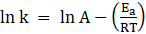⇒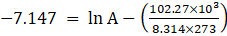(∵ At T = 273K, ln k = -7.147)On solving, we get ln A = 37.911∴ A = 2.91×106When T = 300C, hence T = 30 + 273 = 303K⇒⇒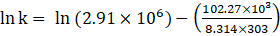⇒ ln k = -2.8⇒ k = 6.08x10-2s-1.When T = 500C, hence T = 50 + 273 = 323KSubstituting in equation 1,A = 2.91 × 106, Ea = 102.27 kJ mol-1Ln K = ln(2.91 × 106)- 102.27/(8.314 × 323)Thus, ln k = -0.5 and k = 0.607s-1.Question 23.The rate constant for the decomposition of hydrocarbons is 2.418 × 10–5s–1 at 546 K. If the energy of activation is 179.9 kJ/mol, what will be the value of pre-exponential factor.Answer:Given,k = 2.418 × 10-5 s-1T = 546 KEa = 179.9 kJ mol-1 = 179.9 × 103J mol-1The Arrhenius equation is given by k = Ae-Ea/RTTaking natural log on both sides,Ln k = ln A-(Ea/RT)Substituting the values,ln(2.418 × 10-5 ) = ln A-179.9/(8.314 × 546)ln A = 12.5917A = 3.9 × 1012 s-1(approximately)Question 24.Consider a certain reaction A → Products with k = 2.0 × 10–2s-1. Calculate the concentration of A remaining after 100 s if the initial concentration of A is 1.0 mol L–1.Answer:Given,k = 2.0 × 10–2s-1time t = 100sConcentration [A0] = 1.0 mol L-1We know,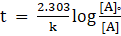On substituting the values,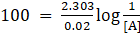Log(1/[A]) = 2.303/2Log[A] = -2.303/2[A] = 0.135 mol L–1Question 25.Sucrose decomposes in acid solution into glucose and fructose according to the first order rate law, with t1/2 = 3.00 hours. What fraction of sample of sucrose remains after 8 hours?Answer:t1/2 = 3.00 hoursWe know, t1/2 = 0.693/k∴ k = 0.693/3k = 0.231hrs-1We know, timeWhere, k- rate constant[R]° -Initial concentration[R]-Concentration at time ‘t’Thus, substituting the values,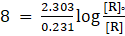log([R]0/[R]) = 0.8log([R]/[R]0) = -0.8[R]/[R]0 = 0.158Hence, 0.158 fraction of sucrose remains.Question 26.The decomposition of hydrocarbon follows the equationk = (4.5 × 1011s–1) e-28000K/T. Calculate Ea.Answer:The given equation isk = (4.5 x 1011 s-1) e-28000 K/T (i)Comparing, Arrhenius equationk = Ae -Ea/RT (ii)We get, Ea / RT = 28000K / T⇒ Ea = R x 28000K= 8.314 J K-1mol-1 × 28000 K= 232792 J mol–1 or 232.792 kJ mol–1Question 27.The rate constant for the first order decomposition of H2O2 is given by the following equation: log k = 14.34 – 1.25 × 104K/TCalculate Ea for this reaction and at what temperature will its half-period be 256 minutes?Answer:We know, The Arrhenius equation is given by k = Ae-Ea/RTTaking natural log on both sides,Ln k = ln A-(Ea/RT)Thus, log k = log A -(Ea/2.303RT) → eqn 1The given equation is log k = 14.34 – 1.25 × 104K/T → eqn 2Comparing 2 equations,Ea/2.303R = 1.25 × 104KEa = 1.25 × 104K × 2.303 × 8.314Ea = 239339.3 J mol-1 (approximately)Ea = 239.34 kJ mol-1Also, when t1/2 = 256 minutes,k = 0.693 / t1/2= 0.693 / 256= 2.707 × 10-3 min-1k = 4.51 × 10-5s–1Substitute k = 4.51 × 10-5s–1 in eqn 2,log 4.51 × 10-5 s–1 = 14.34 – 1.25 × 104K/Tlog(0.654-5) = 14.34– 1.25 × 104K/TT = 1.25 × 104/[ 14.34- log(0.654-5)]T = 668.9K or T = 669 KQuestion 28.The decomposition of A into product has value of k as 4.5 × 103 s–1 at 10°C and energy of activation 60 kJ mol–1. At what temperature would k be 1.5 × 104s–1?Answer:From Arrhenius equation, we obtain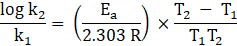Also, k1 = 4.5 × 103 s - 1T1 = 273 + 10 = 283 Kk2 = 1.5 × 104 s - 1Ea = 60 kJ mol - 1 = 6.0 × 104 J mol - 1Then,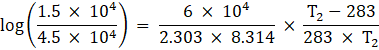→ 0.5229 = 3133.627 × (T2-283)/(283 × T2)→ 0.0472T2 = T2-283T2 = 297K or T2 = 240 CQuestion 29.The time required for 10% completion of a first order reaction at 298K is equal to that required for its 25% completion at 308K. If the value of A is 4 × 1010s–1. Calculate k at 318K and Ea.Answer:We know, time t = (2.303/k) × log([R]0/[R])Where, k- rate constant[R]° -Initial concentration[R]-Concentration at time ‘t’At 298K, If 10% is completed, then 90% is remaining.t = (2.303/k) × log ([R]0/0.9[R]0)t = (2.303/k) × log (1/0.9)t = 0.1054 / kAt temperature 308K, 25% is completed, 75% is remainingt’ = (2.303/k’) × log ([R]0/0.75[R]0)t’ = (2.303/k’) × log (1/0.75)t’ = 2.2877 / k'But, t = t’0.1054 / k = 2.2877 / k'k' / k = 2.7296From Arrhenius equation, we obtainlog k2/k1 = (Ea / 2.303 R) × (T2 - T1) / T1T2Substituting the values,⇒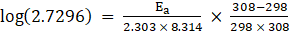Ea = 76640.09 J mol-1 or 76.64 kJ mol-1We know, log k = log A –Ea/RTLog k = log(4 × 1010)-(76.64kJ mol-1/(8.314 × 318))Log k = -1.986∴ k = 1.034 x 10-2 s -1Question 30.The rate of a reaction quadruples when the temperature changes from 293 K to 313 K. Calculate the energy of activation of the reaction assuming that it does not change with temperature.Answer:Given, k2 = 4k1, T1 = 293K and T2 = 313KWe know, From Arrhenius equation, we obtain⇒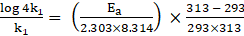⇒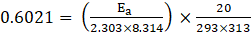On solving we get,Ea = 58263.33 J mol-1 or 58.26 kJ mol-1

PDF FILE TO YOUR EMAIL IMMEDIATELY PURCHASE NOTES & PAPER SOLUTION. @ Rs. 50/- each (GST extra)

HINDI ENTIRE PAPER SOLUTION

MARATHI PAPER SOLUTION

SSC MATHS I PAPER SOLUTION

SSC MATHS II PAPER SOLUTION

SSC SCIENCE I PAPER SOLUTION

SSC SCIENCE II PAPER SOLUTION

SSC ENGLISH PAPER SOLUTION

SSC & HSC ENGLISH WRITING SKILL

HSC ACCOUNTS NOTES

HSC OCM NOTES

HSC ECONOMICS NOTES

HSC SECRETARIAL PRACTICE NOTES

2019 Board Paper Solution

HSC ENGLISH SET A 2019 21st February, 2019

HSC ENGLISH SET B 2019 21st February, 2019

HSC ENGLISH SET C 2019 21st February, 2019

HSC ENGLISH SET D 2019 21st February, 2019

SECRETARIAL PRACTICE (S.P) 2019 25th February, 2019

HSC XII PHYSICS 2019 25th February, 2019

CHEMISTRY XII HSC SOLUTION 27th, February, 2019

OCM PAPER SOLUTION 2019 27th, February, 2019

HSC MATHS PAPER SOLUTION COMMERCE, 2nd March, 2019

HSC MATHS PAPER SOLUTION SCIENCE 2nd, March, 2019

SSC ENGLISH STD 10 5TH MARCH, 2019.

HSC XII ACCOUNTS 2019 6th March, 2019

HSC XII BIOLOGY 2019 6TH March, 2019

HSC XII ECONOMICS 9Th March 2019

SSC Maths I March 2019 Solution 10th Standard11th, March, 2019

SSC MATHS II MARCH 2019 SOLUTION 10TH STD.13th March, 2019

SSC SCIENCE I MARCH 2019 SOLUTION 10TH STD. 15th March, 2019.

SSC SCIENCE II MARCH 2019 SOLUTION 10TH STD. 18th March, 2019.

SSC SOCIAL SCIENCE I MARCH 2019 SOLUTION20th March, 2019

SSC SOCIAL SCIENCE II MARCH 2019 SOLUTION, 22nd March, 2019

XII CBSE - BOARD - MARCH - 2019 ENGLISH - QP + SOLUTIONS, 2nd March, 2019

HSC Maharashtra Board Papers 2020

(Std 12th English Medium)

HSC ECONOMICS MARCH 2020

HSC OCM MARCH 2020

HSC ACCOUNTS MARCH 2020

HSC S.P. MARCH 2020

HSC ENGLISH MARCH 2020

HSC HINDI MARCH 2020

HSC MARATHI MARCH 2020

HSC MATHS MARCH 2020

SSC Maharashtra Board Papers 2020

(Std 10th English Medium)

English MARCH 2020

HindI MARCH 2020

Hindi (Composite) MARCH 2020

Marathi MARCH 2020

Mathematics (Paper 1) MARCH 2020

Mathematics (Paper 2) MARCH 2020

Sanskrit MARCH 2020

Sanskrit (Composite) MARCH 2020

Science (Paper 1) MARCH 2020

Science (Paper 2)

MUST REMEMBER THINGS on the day of Exam

Are you prepared? for English Grammar in Board Exam.

Paper Presentation In Board Exam

How to Score Good Marks in SSC Board Exams

Tips To Score More Than 90% Marks In 12th Board Exam

How to write English exams?

How to prepare for board exam when less time is left

How to memorise what you learn for board exam

No. 1 Simple Hack, you can try out, in preparing for Board Exam

How to Study for CBSE Class 10 Board Exams Subject Wise Tips?

JEE Main 2020 Registration Process – Exam Pattern & Important Dates

NEET UG 2020 Registration Process Exam Pattern & Important Dates

How can One Prepare for two Competitive Exams at the same time?

8 Proven Tips to Handle Anxiety before Exams!